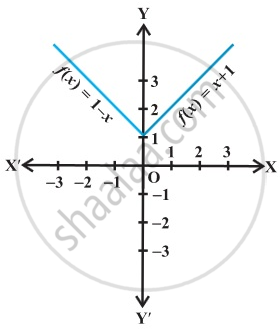Department of Pre-University Education, KarnatakaPUC Karnataka Science Class 11
Advertisement Remove all ads

# The Function F is Defined by F ( X ) = ⎧ ⎨ ⎩ 1 − X , X < 0 1 , X = 0 X + 1 , X > 0 . Draw the Graph of F(X). - Mathematics

The function f is defined by

$f\left( x \right) = \begin{cases}1 - x, & x < 0 \\ 1 , & x = 0 \\ x + 1, & x > 0\end{cases}$ . Draw the graph of f(x).

Advertisement Remove all ads

#### Solution

Here,
f (x) = 1 – x for x < 0. So,
(-4) = 1 – ( -4) = 5
f (- 3) = 1 – ( -3) = 4
f (-2) = 1 – ( -2) = 3
( -1) = 1 – ( -1) = 2 etc.

Also, f(x) = 1 for x = 0.

Lastly,  f (x) = x + 1 for, x > 0.
and f (1) = 2, f (2) = 3, f (3) = 4, f (4) = 5 and so on.

Thus, the graph of f is as shown below:Is there an error in this question or solution?
Advertisement Remove all ads

#### APPEARS IN

RD Sharma Class 11 Mathematics Textbook
Chapter 3 Functions
Exercise 3.4 | Q 7 | Page 38
Advertisement Remove all ads

#### Video TutorialsVIEW ALL 

Advertisement Remove all ads
Share
Notifications

View all notifications

Forgot password?